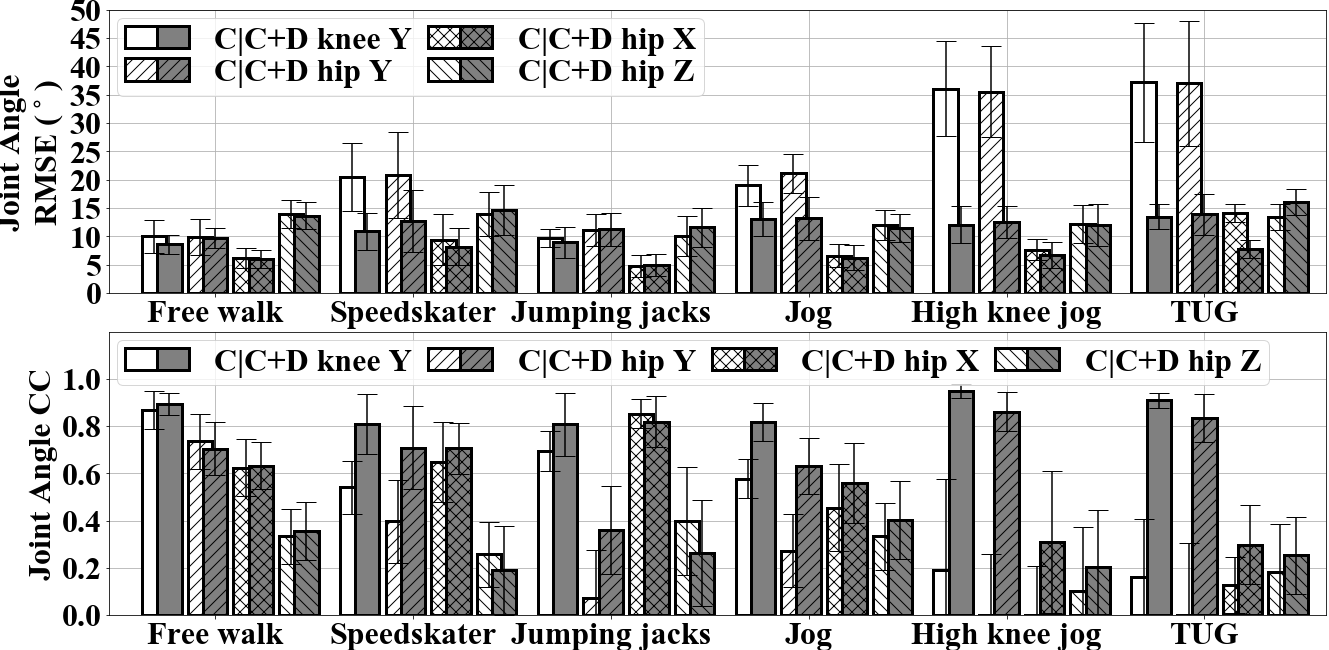# Estimating Lower Limb Kinematics using Distance Measurements with a Reduced Wearable Inertial Sensor Count

Luke Wicent Sy, Nigel Lovell, Stephen Redmond

## Gait Analysis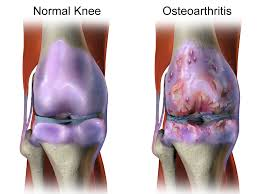Osteoarthritis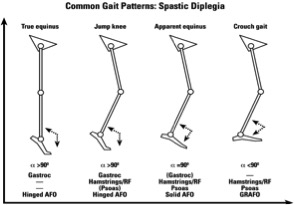Cerebral Palsy Surgery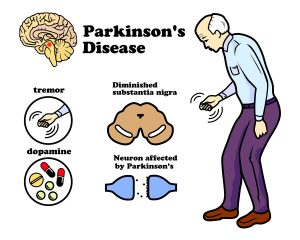Parkinson's Disease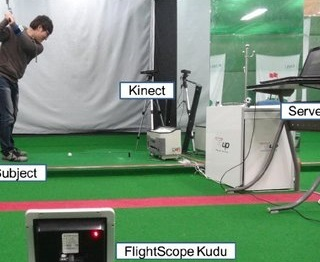Perform. Improvement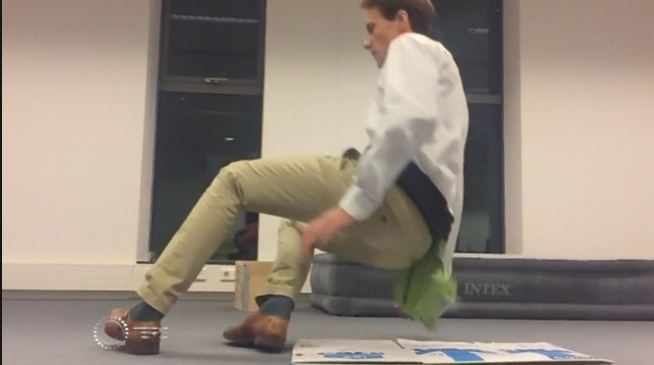Fall risk assessment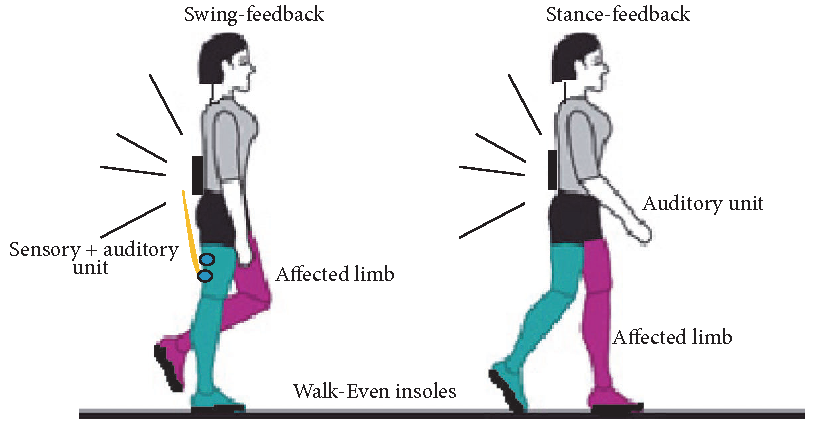Real Time Feedback

## Motion Capture Systems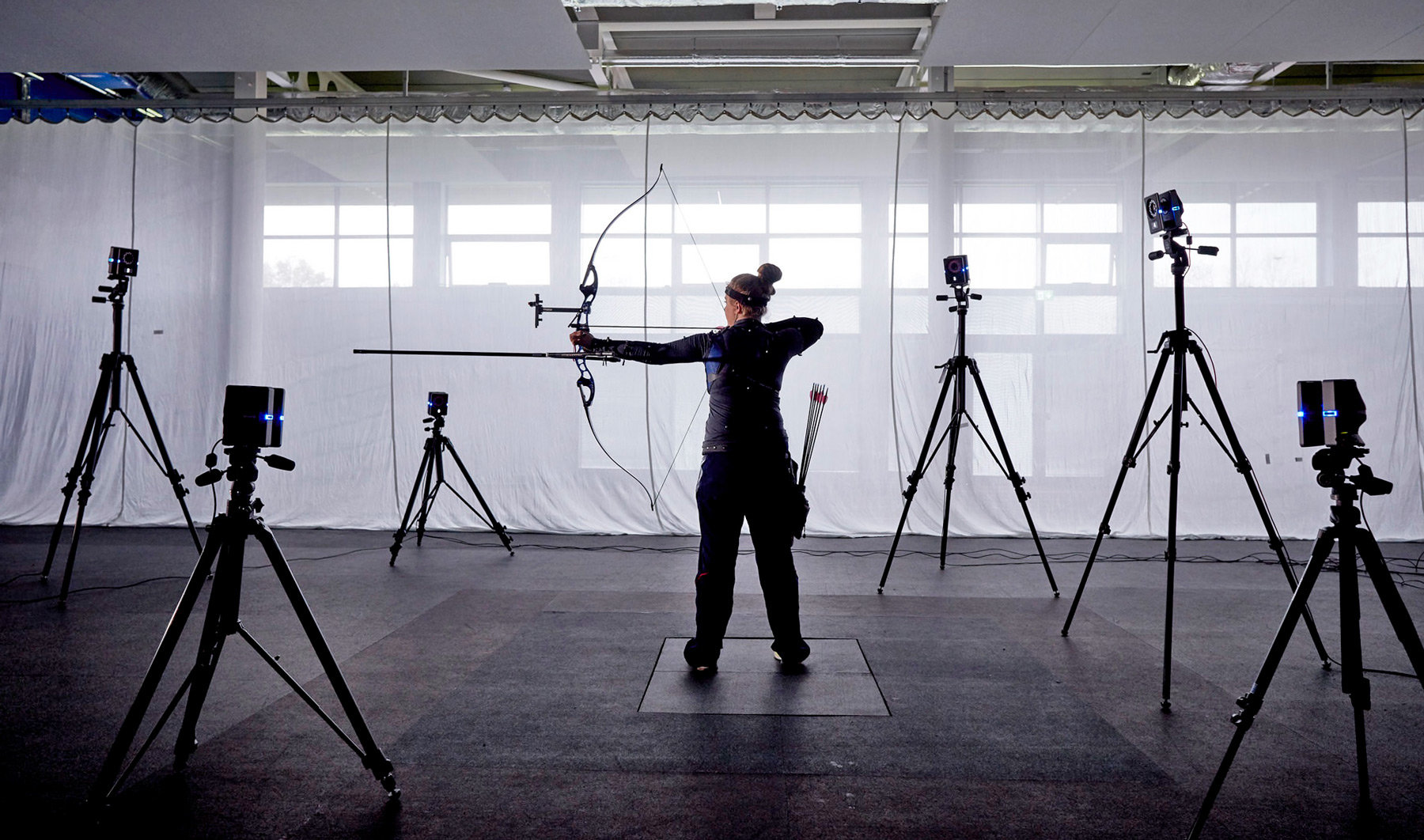#### Camera based

Very accurate but limited to a small space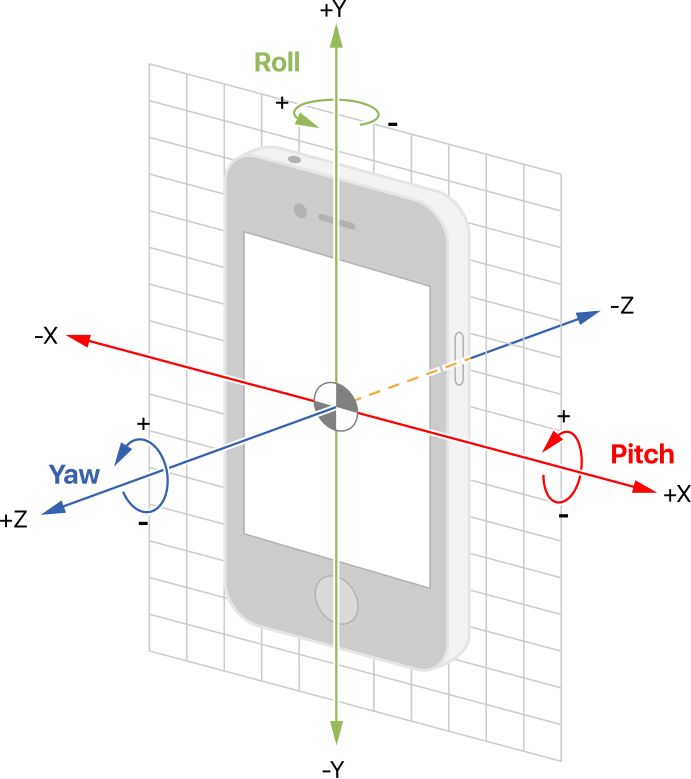#### Inertial Measurement Unit (IMU)

Miniaturization. Track position and orientation (albeit with drift).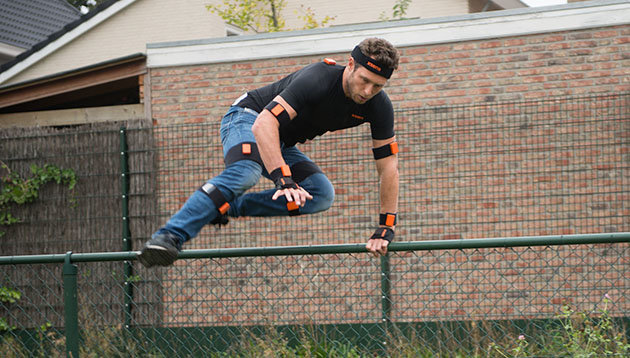#### IMU based (one sensor per seg)

Can capture almost everywhere. Can be conspicuous for everyday use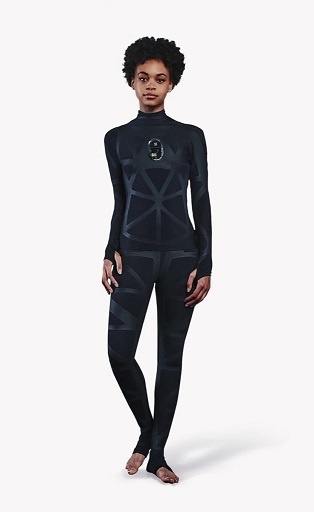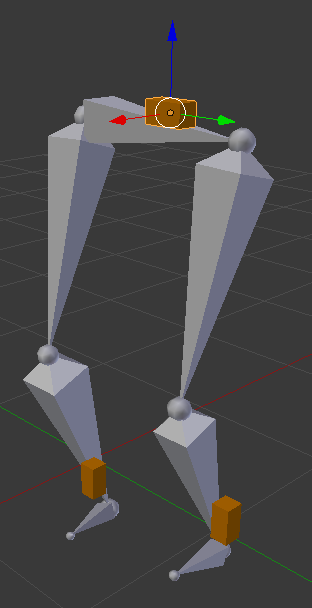#### Wearable based

More comfortable

• Soft stretch sensors
• More & smaller IMUs
• Sparse sensors

#### Wearable based

More comfortable

• Soft stretch sensors
• More & smaller IMUs
• Sparse sensors

## Sparse Wearable Challenge

Goal: Comfortable, Fast, and Accurate Motion Capture System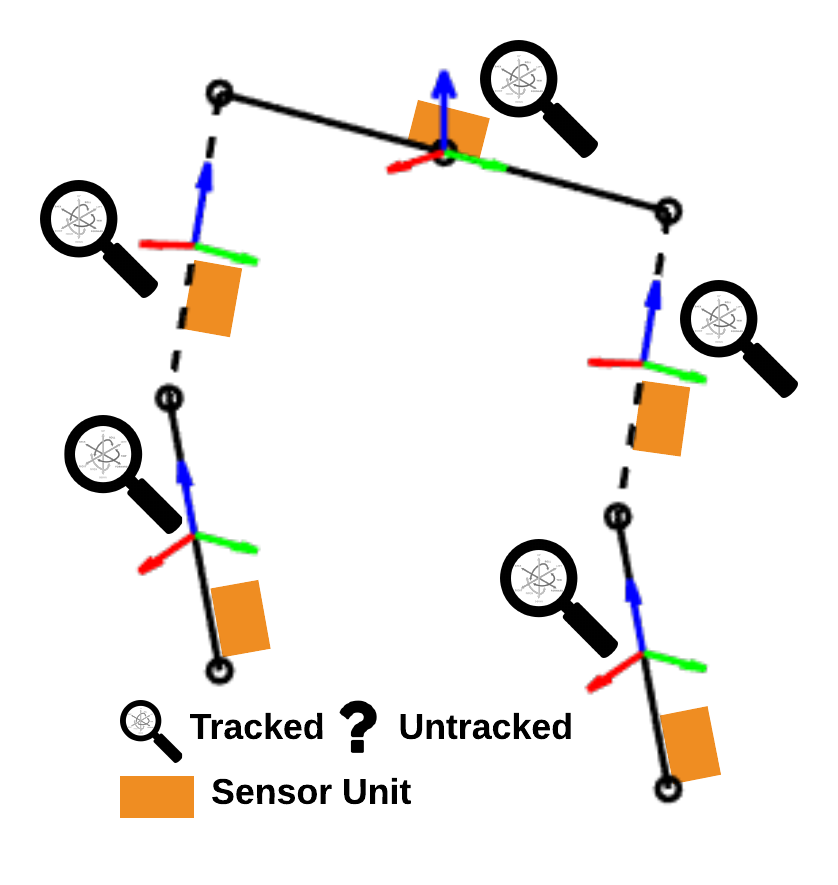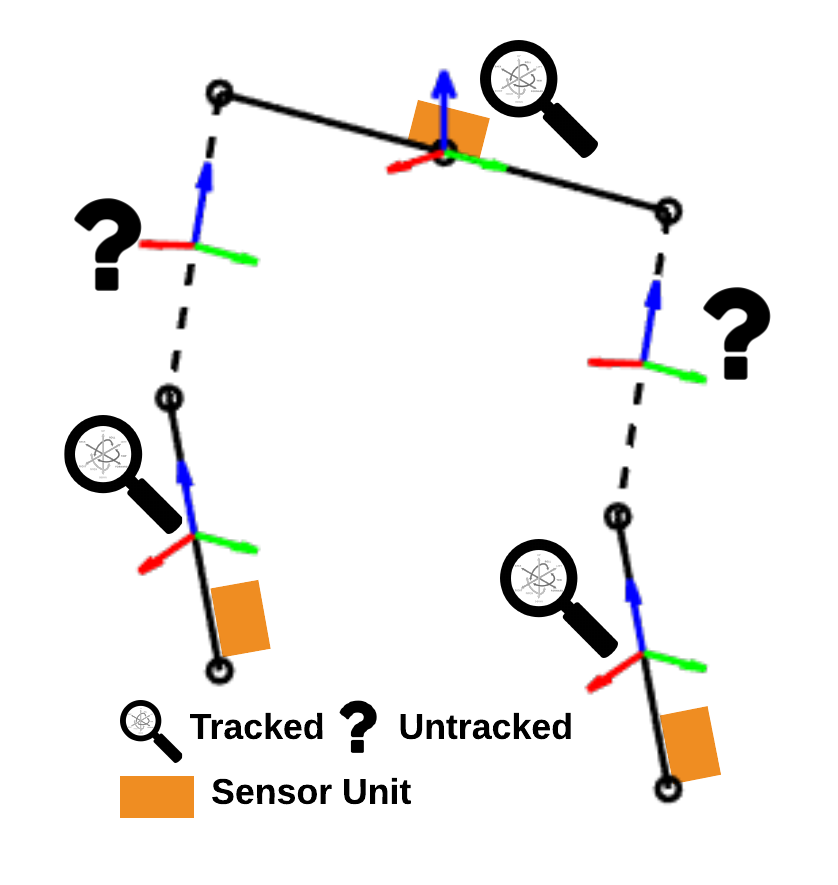One sensor per segment.

Less sensor = Missing info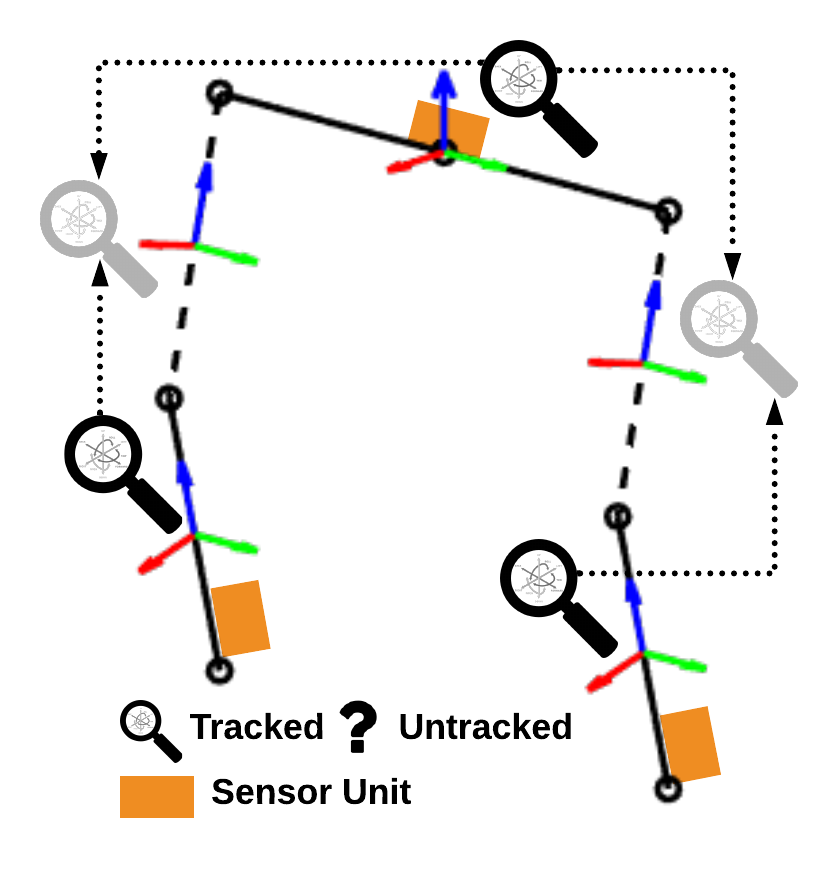Infer through biomechanical constraints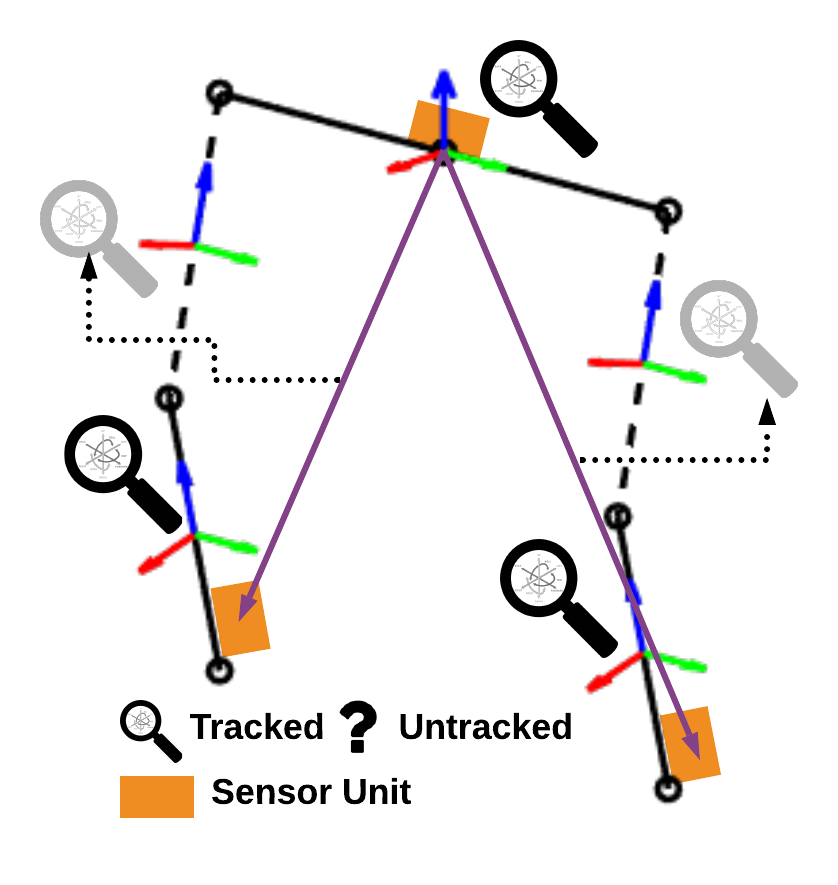## Sparse Constrained KF (CKF)

### Algorithm overview of prior work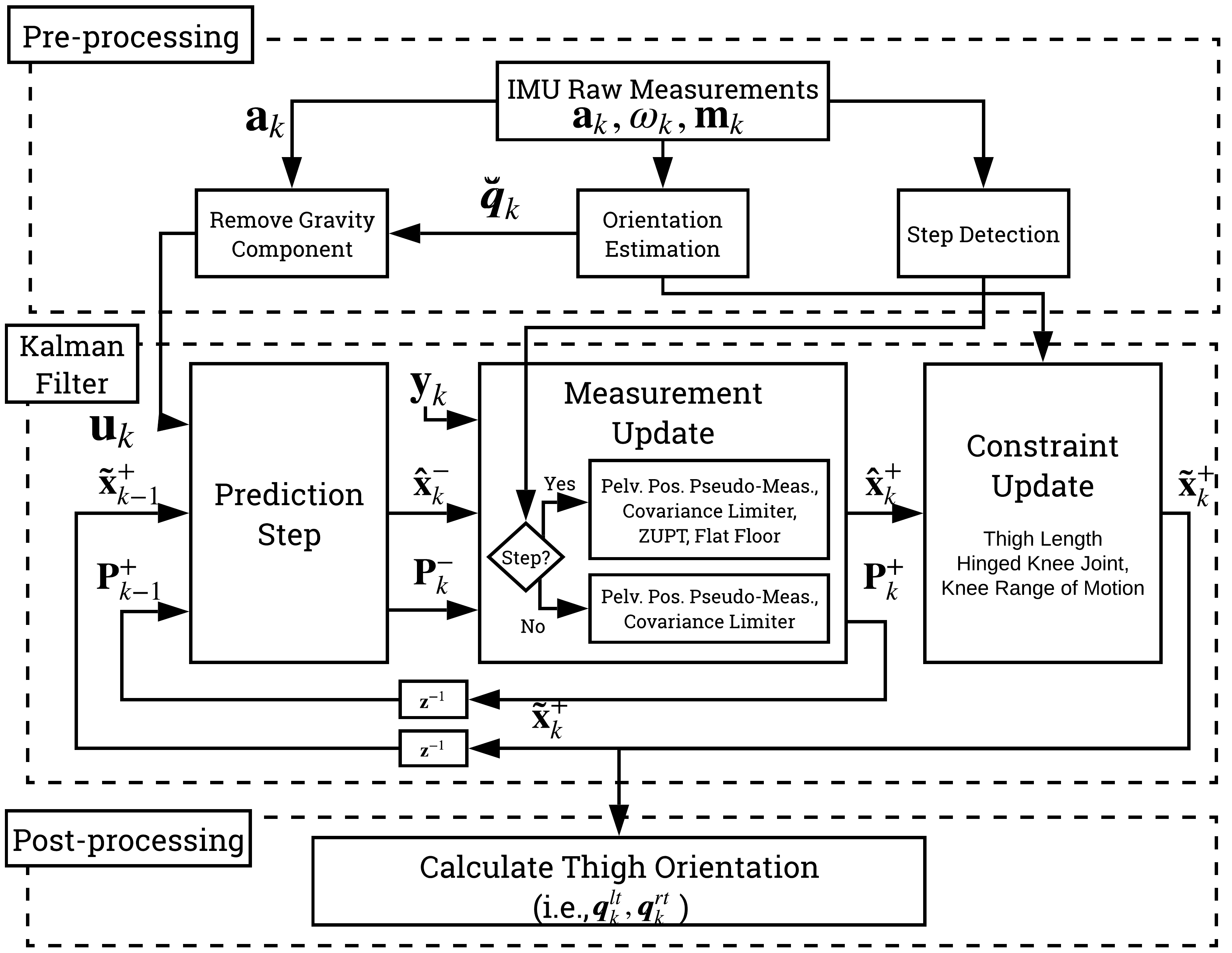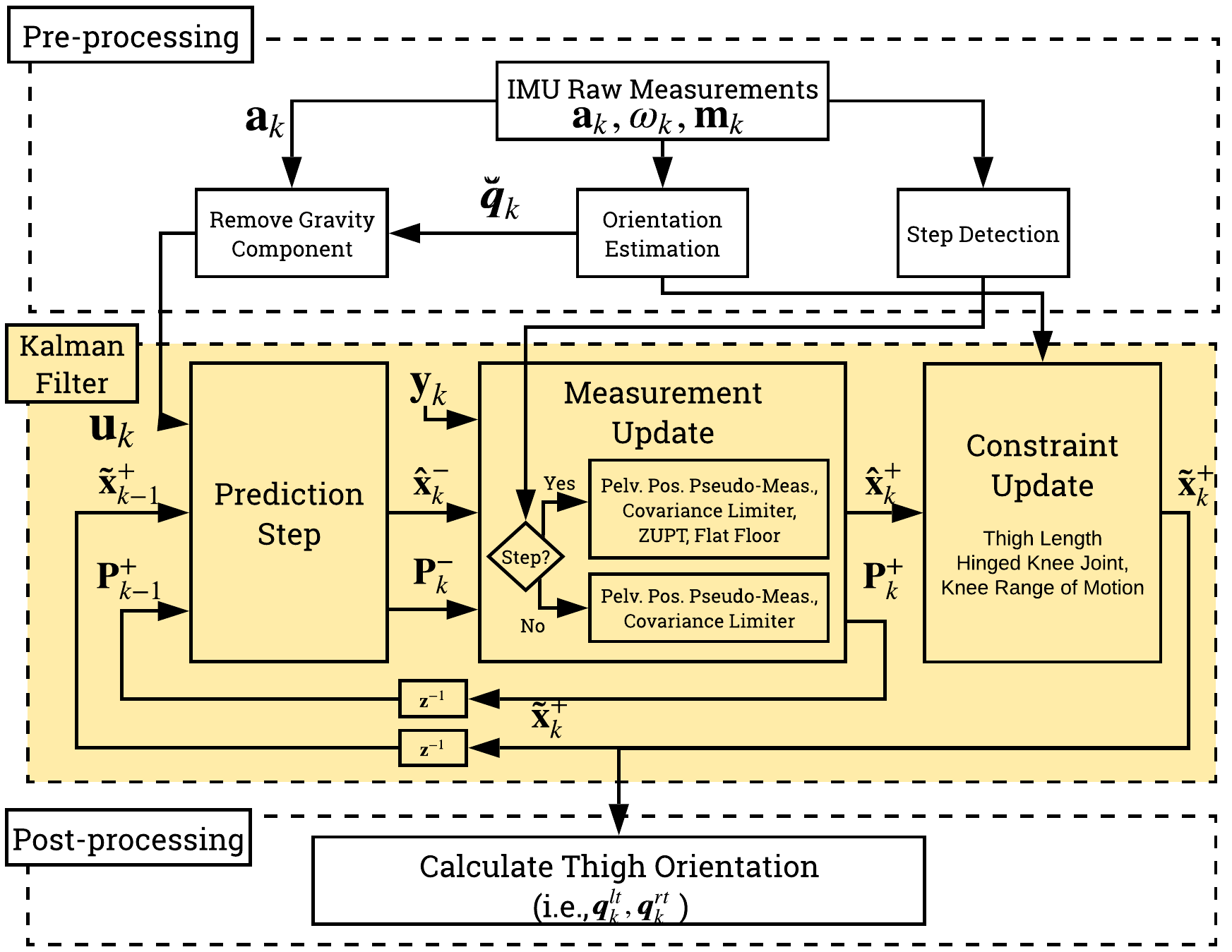#### Body Model: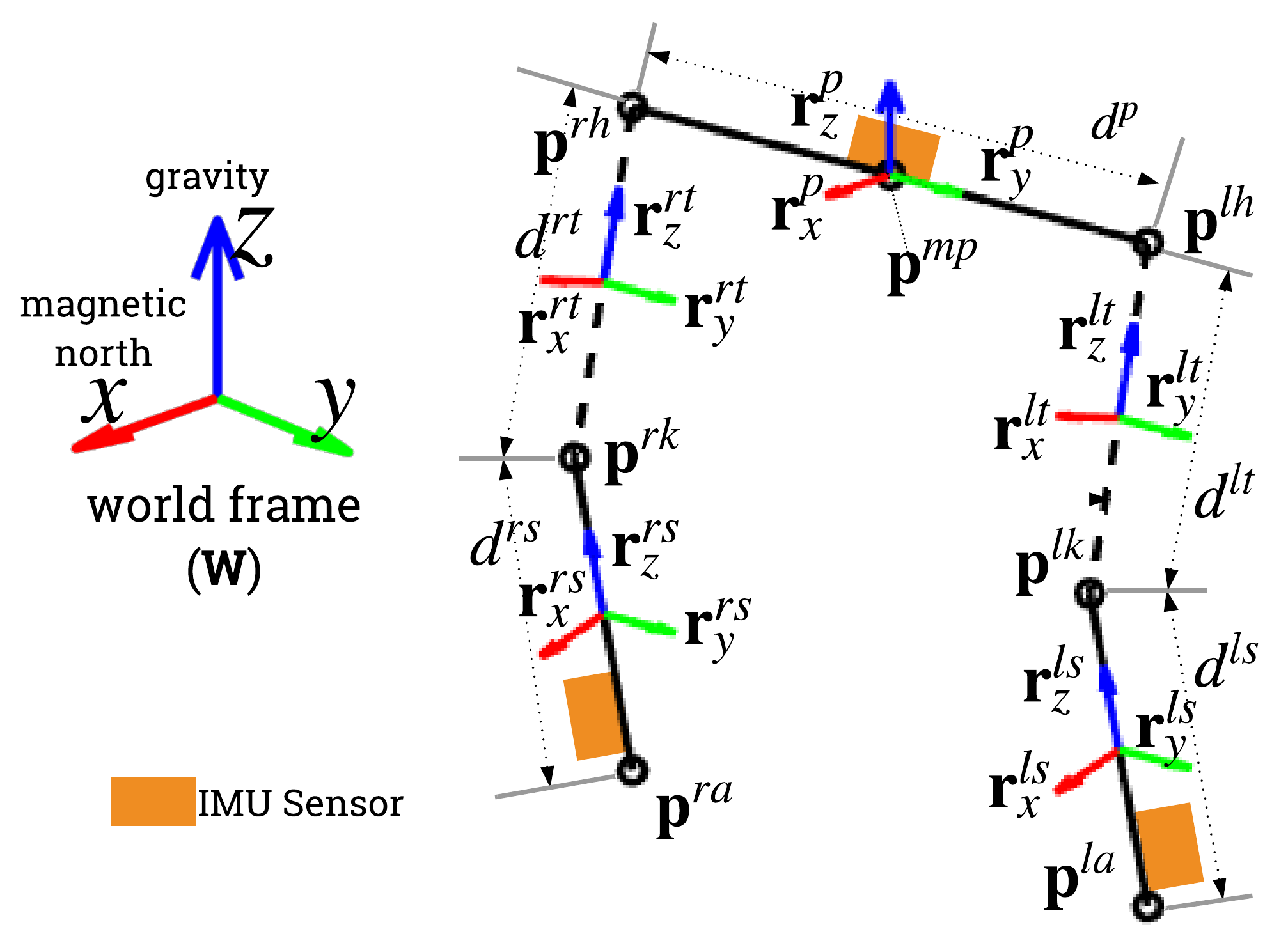Pred.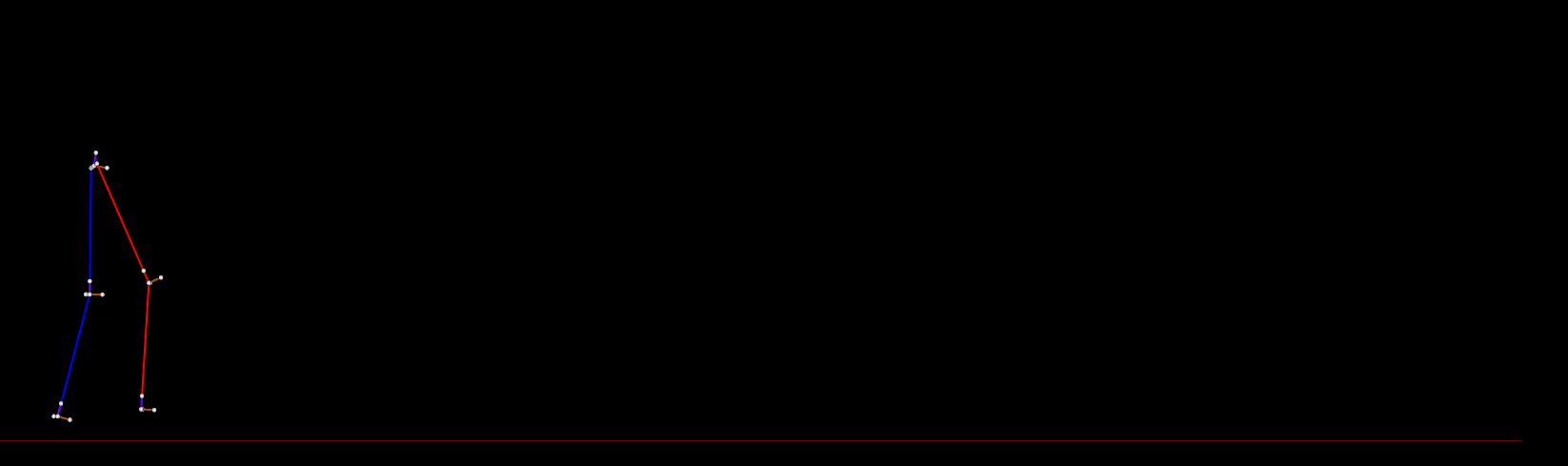Meas.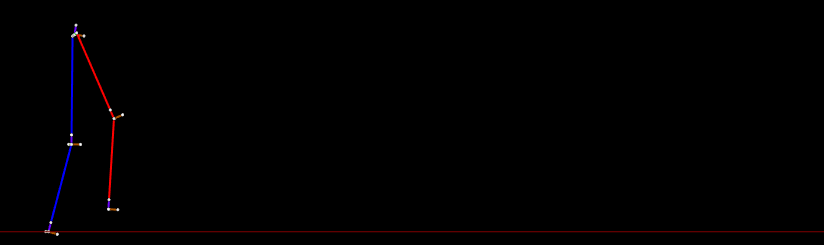Cstr.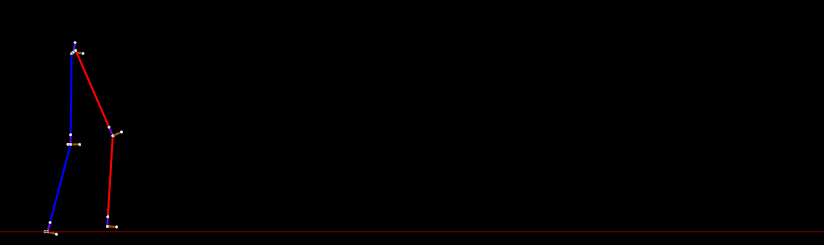## Sparse CKF - Sample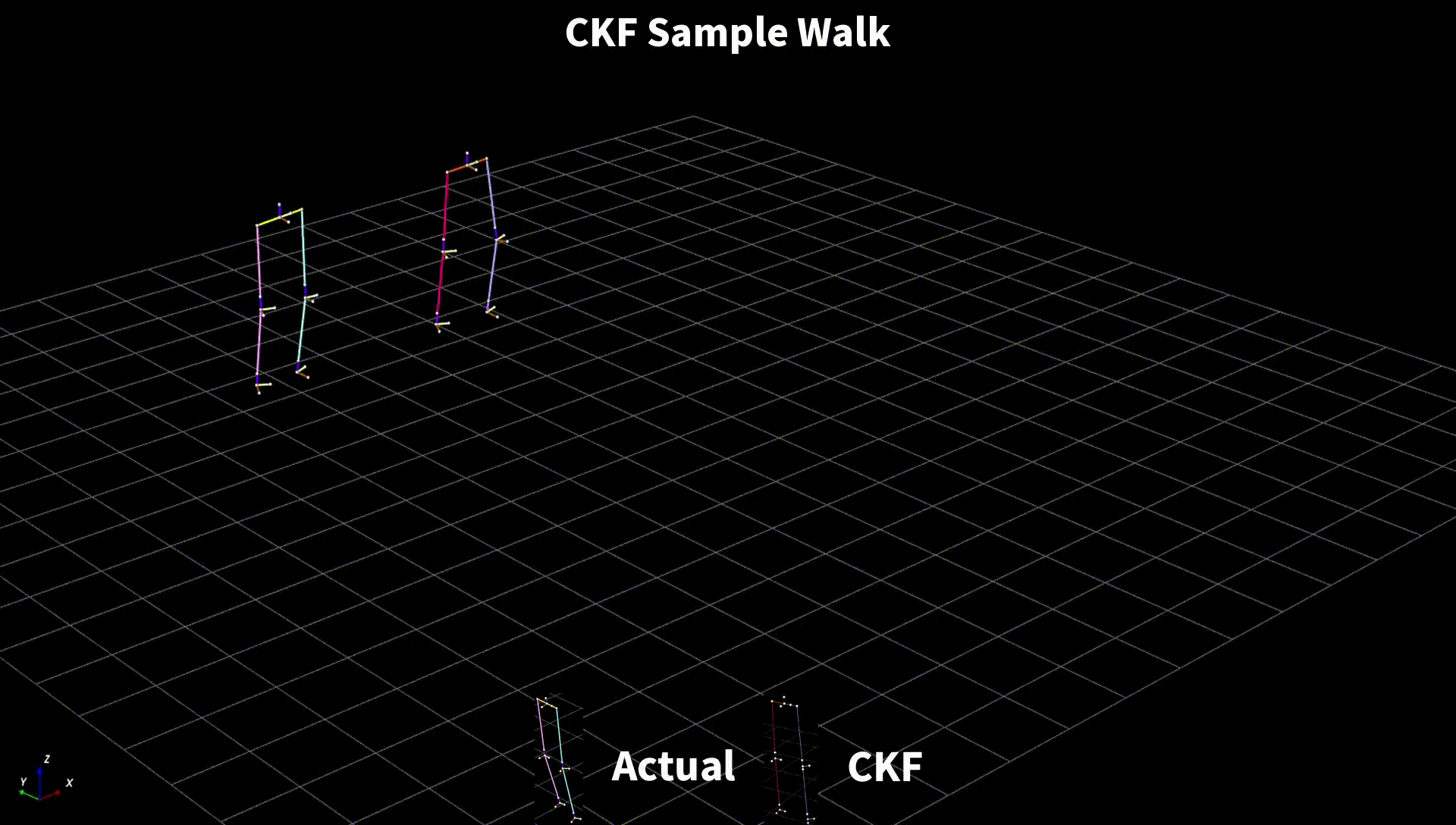## Sparse CKF - Weakness

To make it work, we need to make assumptions that may not be practical for certain movements (e.g., Activities of Daily Living or ADLs).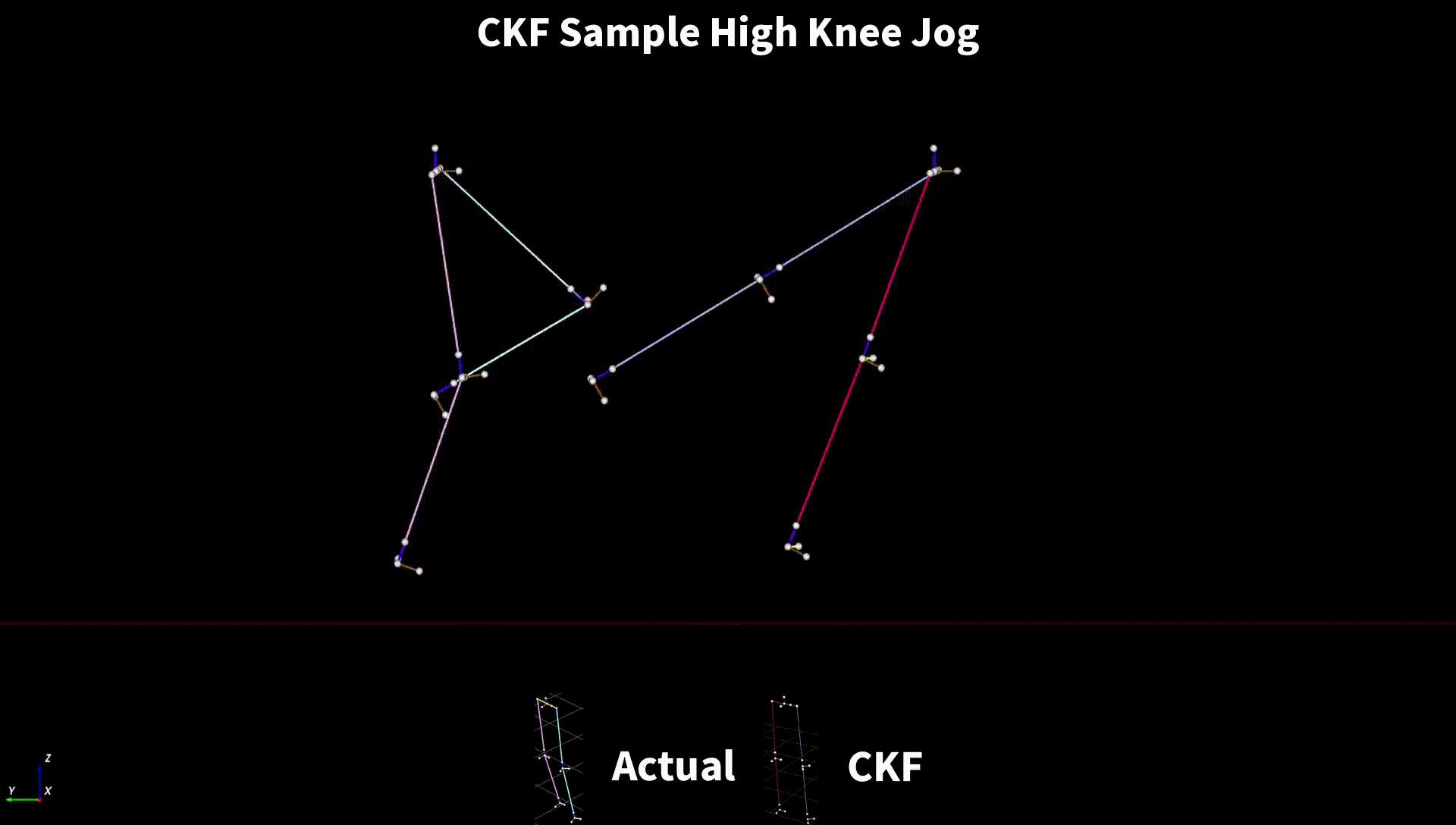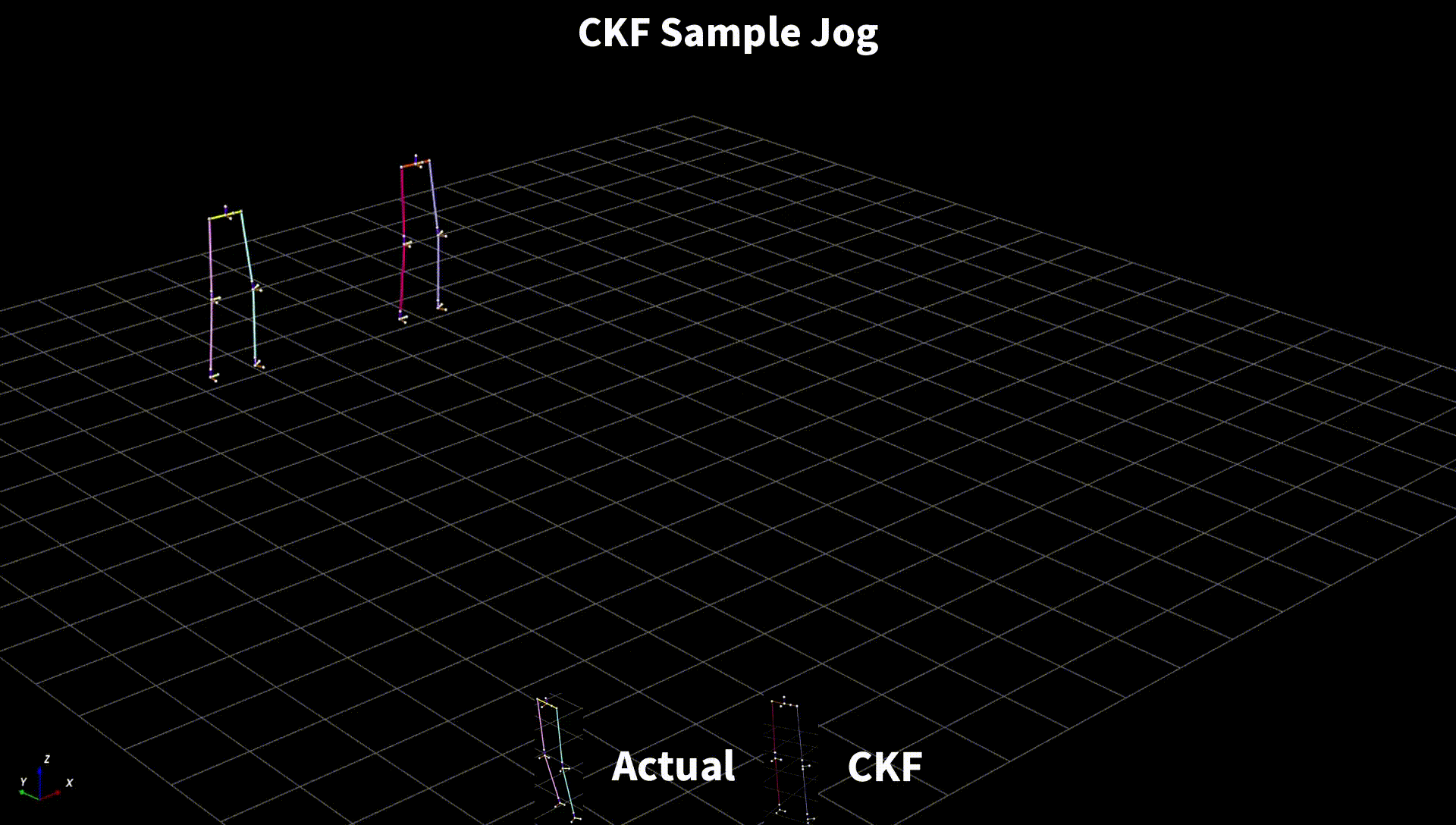## Sparse CKF + Distance

### Overview

Distance measurement which can be obtained through ultrasonic or ultra-wide band radio (UWB).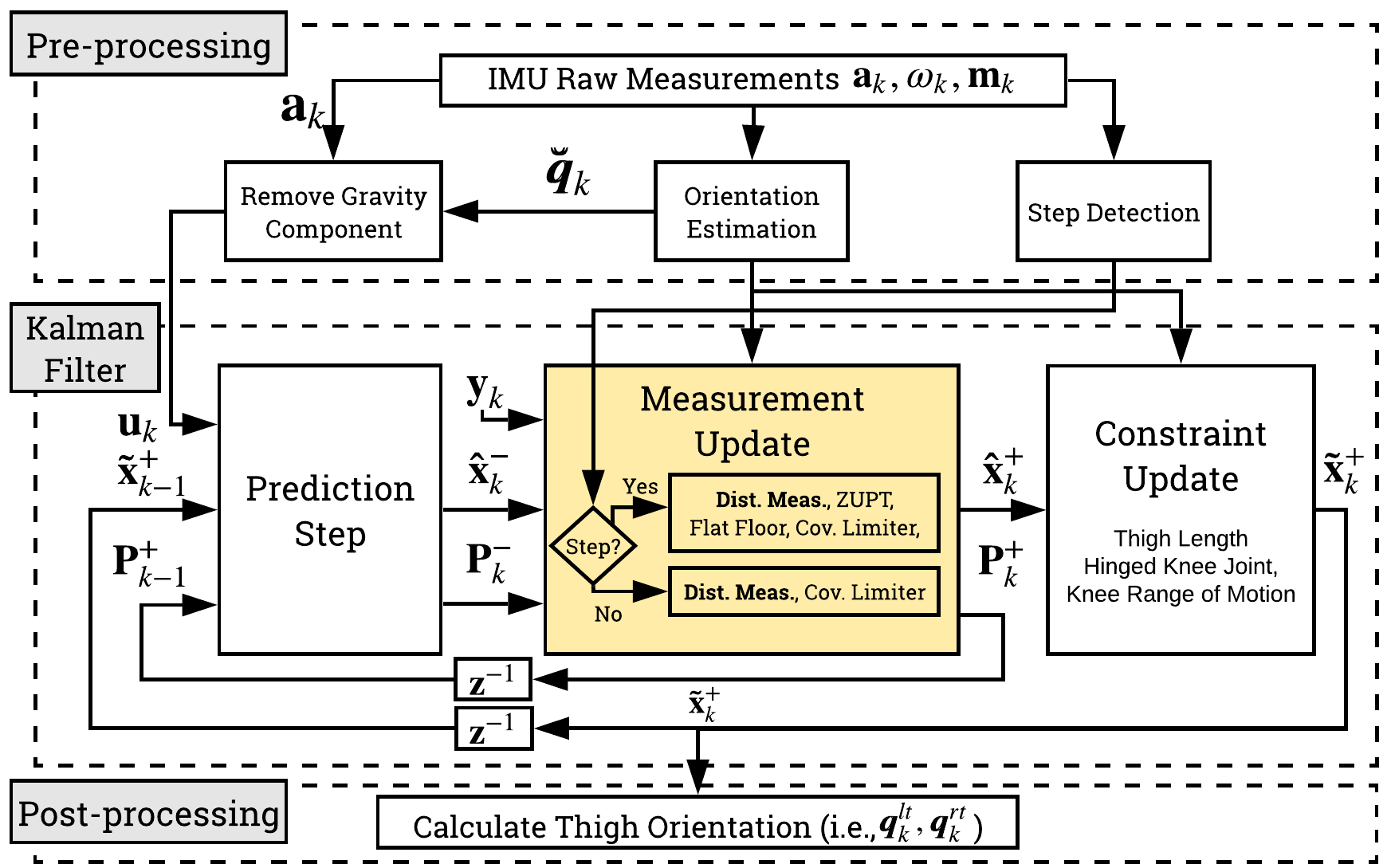#### Body Model: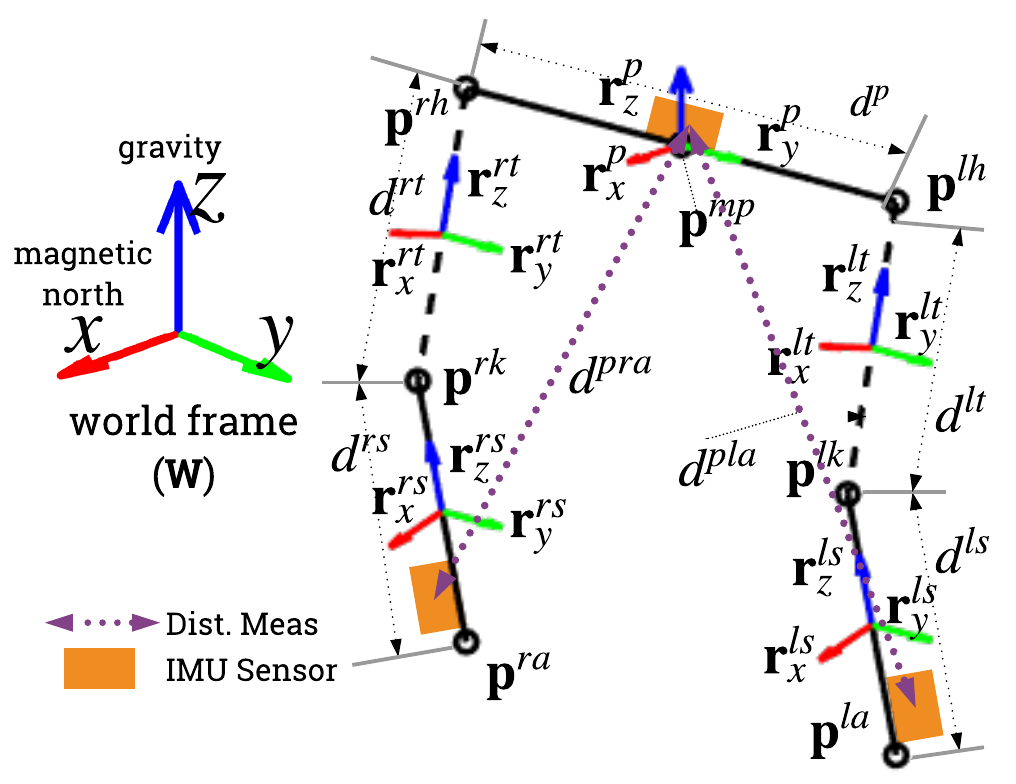## Sparse CKF + Distance

Remove pelvis related assumptions. Additional measurement model.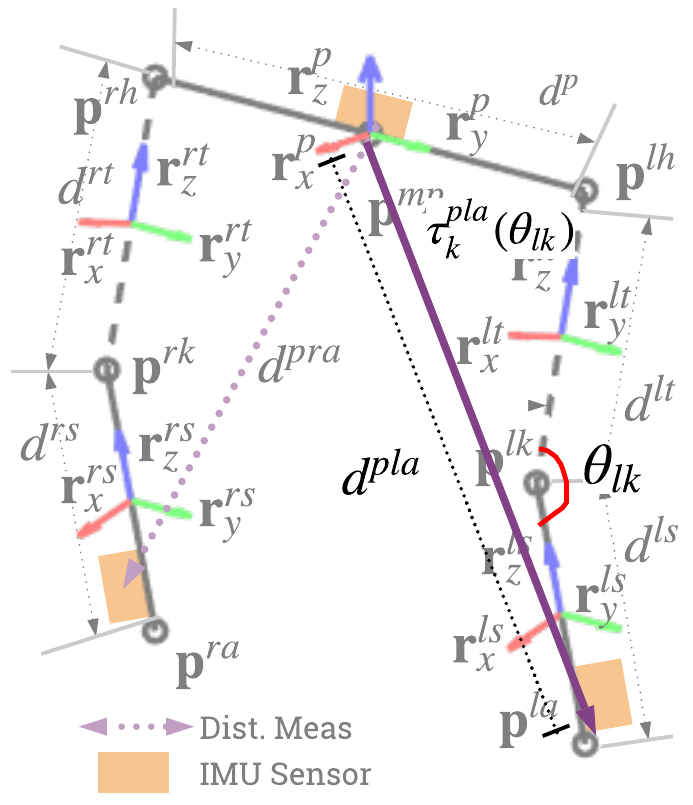$$\mathbf{H}(\mathbf{x}) = \tau_{k}^{pla} ( \hat{\theta}_{lk} ) \\ \tau_{k}^{pla} ( \theta_{lk} ) = \overbrace{\tfrac{d^{p}}{2} \mathbf{r}^p_y - d^{ls} \mathbf{r}^{ls}_{z}}^{\psi, \text{ hip + shanks}} + \overbrace{d^{lt} \mathbf{r}_x^{ls} \sin{(\theta_{lk})} -d^{lt} \mathbf{r}^{ls}_z \cos{(\theta_{lk})} }^{\Lambda, \text{ thigh}} \\ (\hat{d}^{pla})^2 = \tau_{k}^{pla}(\theta_{lk})^2 = \psi^2 + 2 \psi \cdot \Lambda + (d^{lt})^2 \\ \alpha \cos{(\theta_{lk})} + \beta \sin{(\theta_{lk})} = \gamma \\ \alpha = - 2 d^{lt} \psi \cdot \mathbf{r}^{ls}_z, \quad \beta = 2 d^{lt} \psi \cdot \mathbf{r}^{ls}_x \\ \gamma = (\hat{d}^{pla})^2 - \psi^2 - (d^{lt})^2 \\ \hat{\theta}_{lk} = \cos^{-1}\left( \tfrac{\alpha \gamma \pm \beta \sqrt{\alpha^2+\beta^2-\gamma^2}}{\alpha^2+\beta^2} \right)$$

## Sparse CKF+D - Sample

Tested on actual IMU data + simulated distance measurement from Sparse CKF dataset (walking, jumping jacks, speedskater, TUG, jog). Most deviation is at the turning motion ($t=3.5 - 5$s).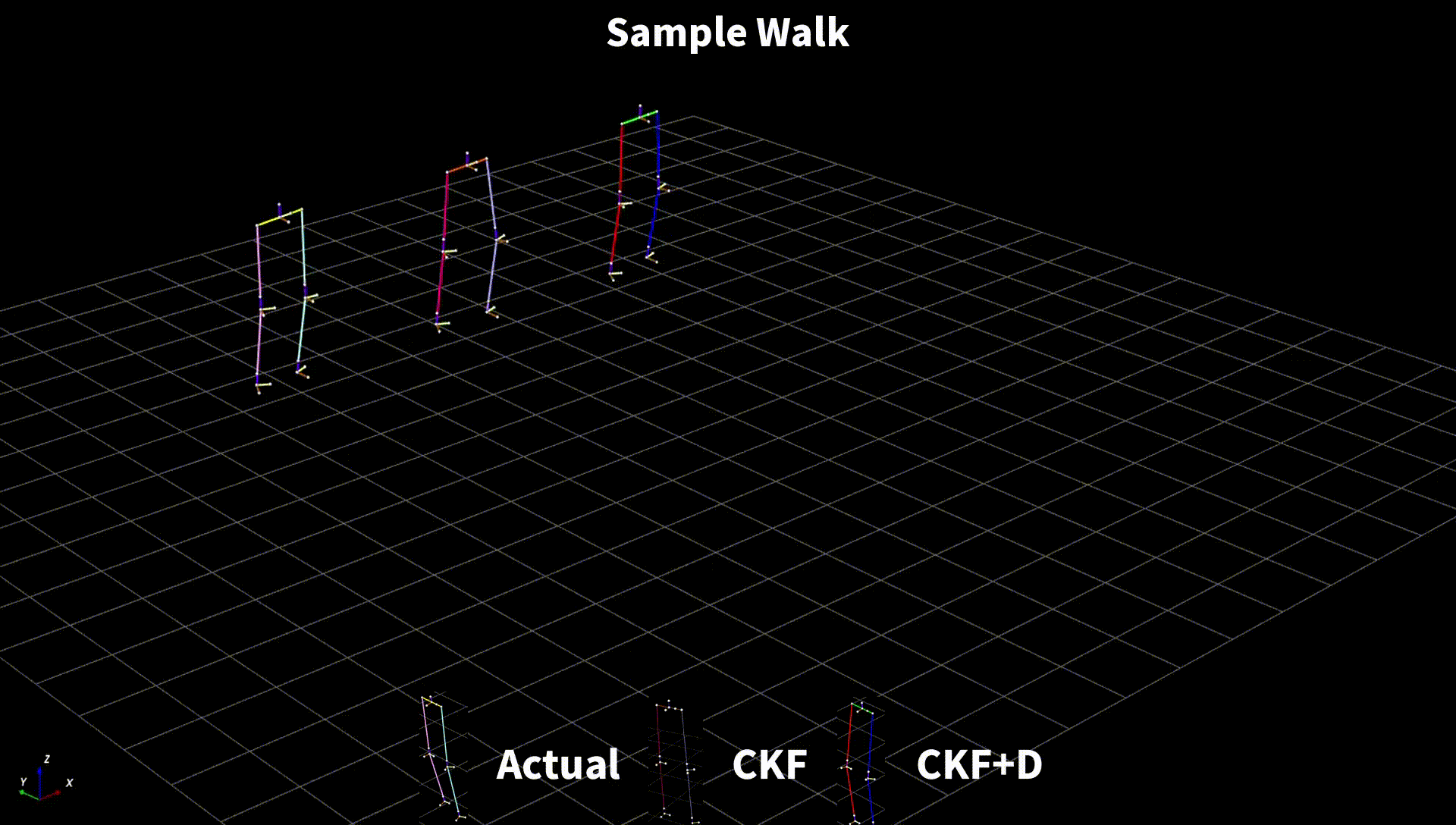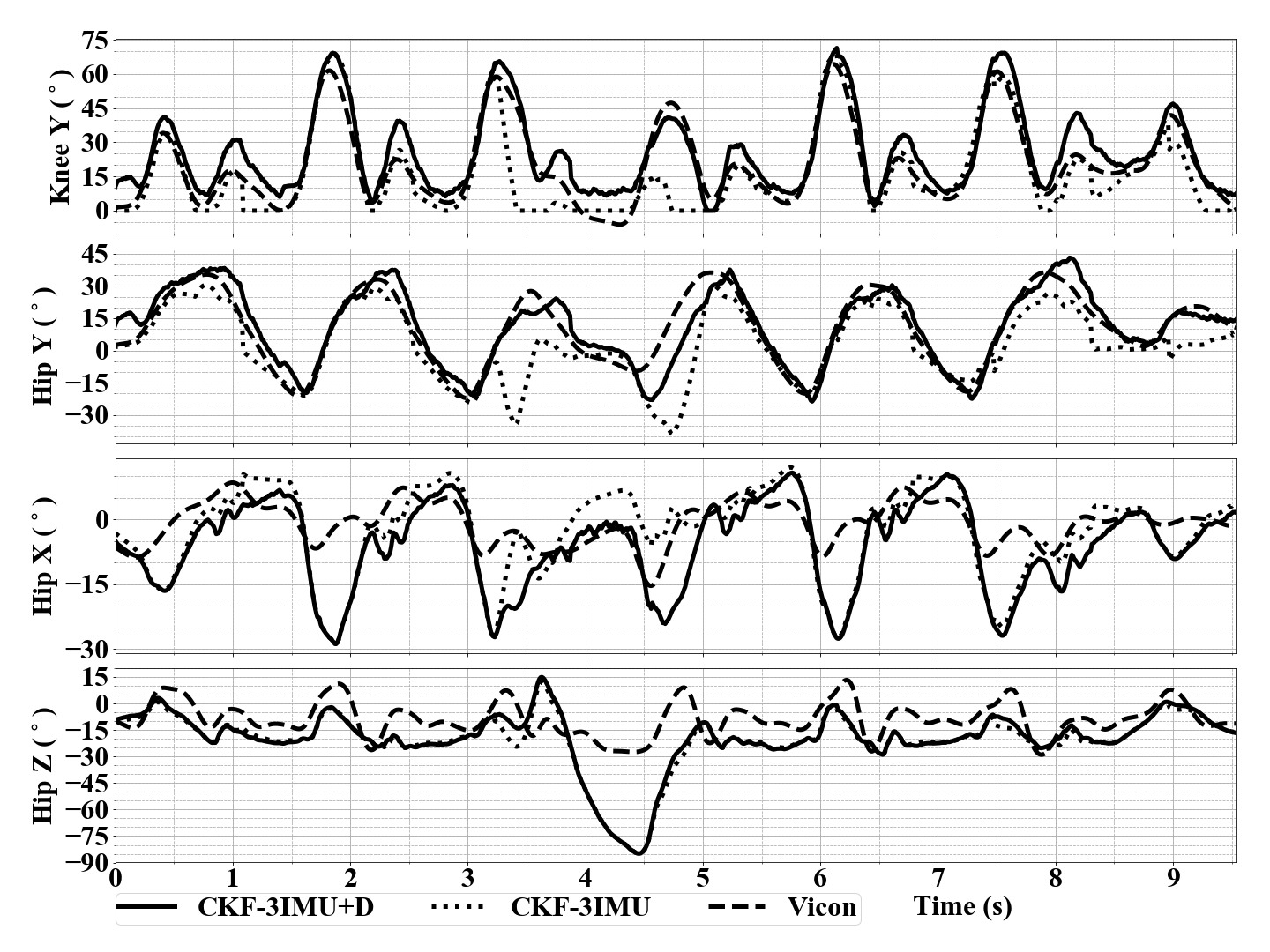## Sparse CKF+D - Sample

Dramatic increase in performance in dynamic movements. Captures Sagitall knee angles better.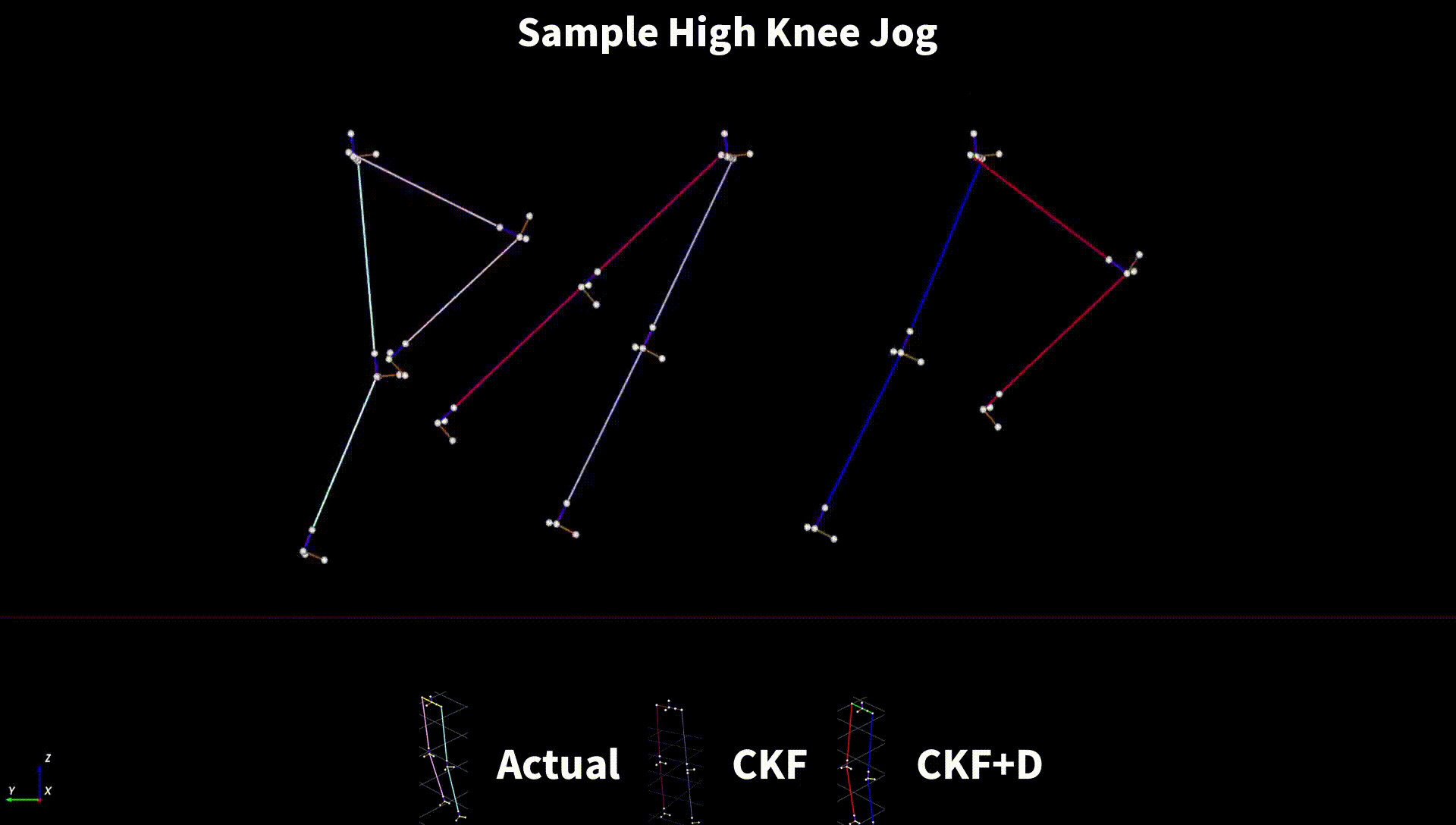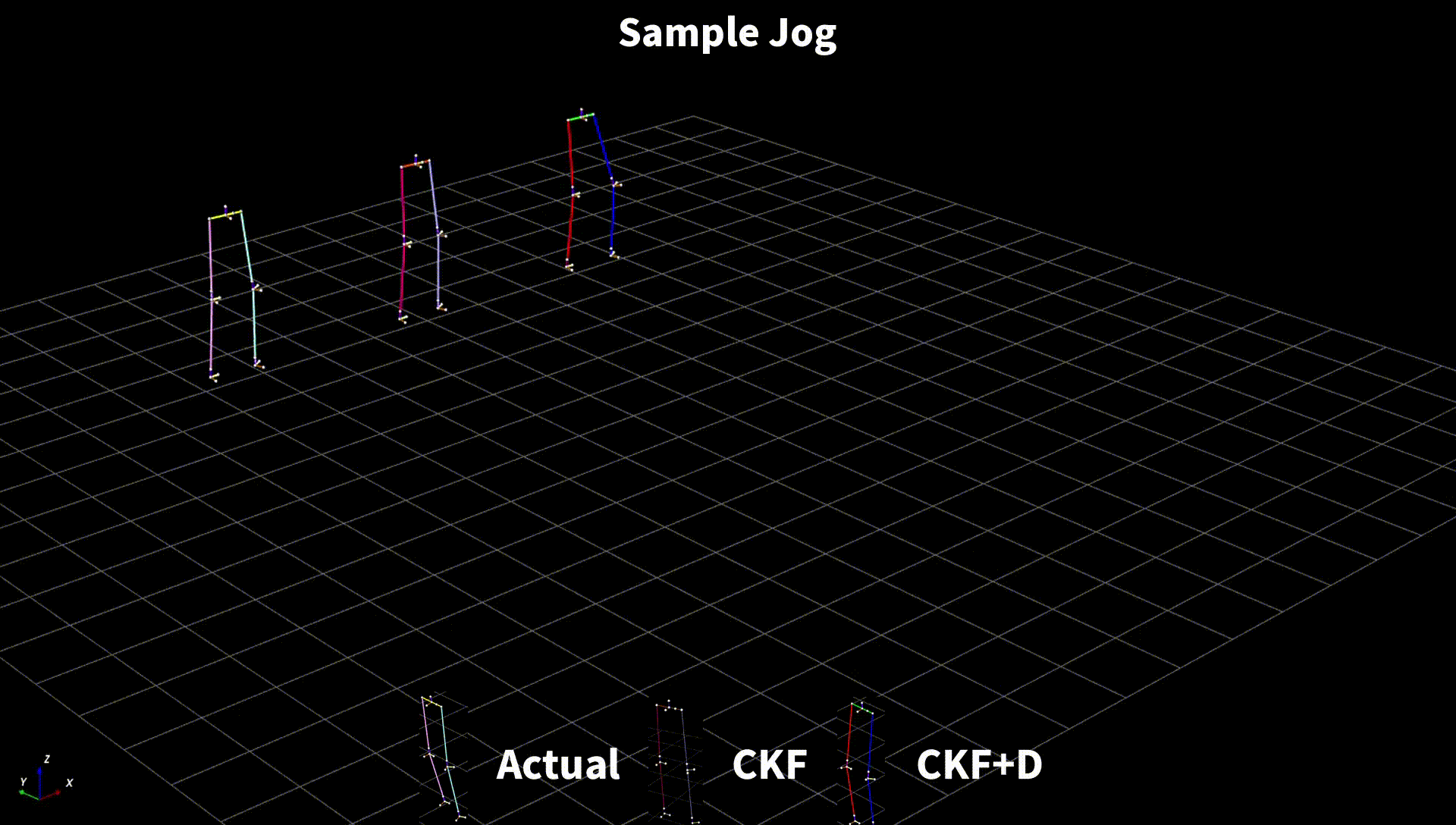## Sparse CKF+D - Sample

Is able to locate relative position better. Note: TUG = Timed Up and Go.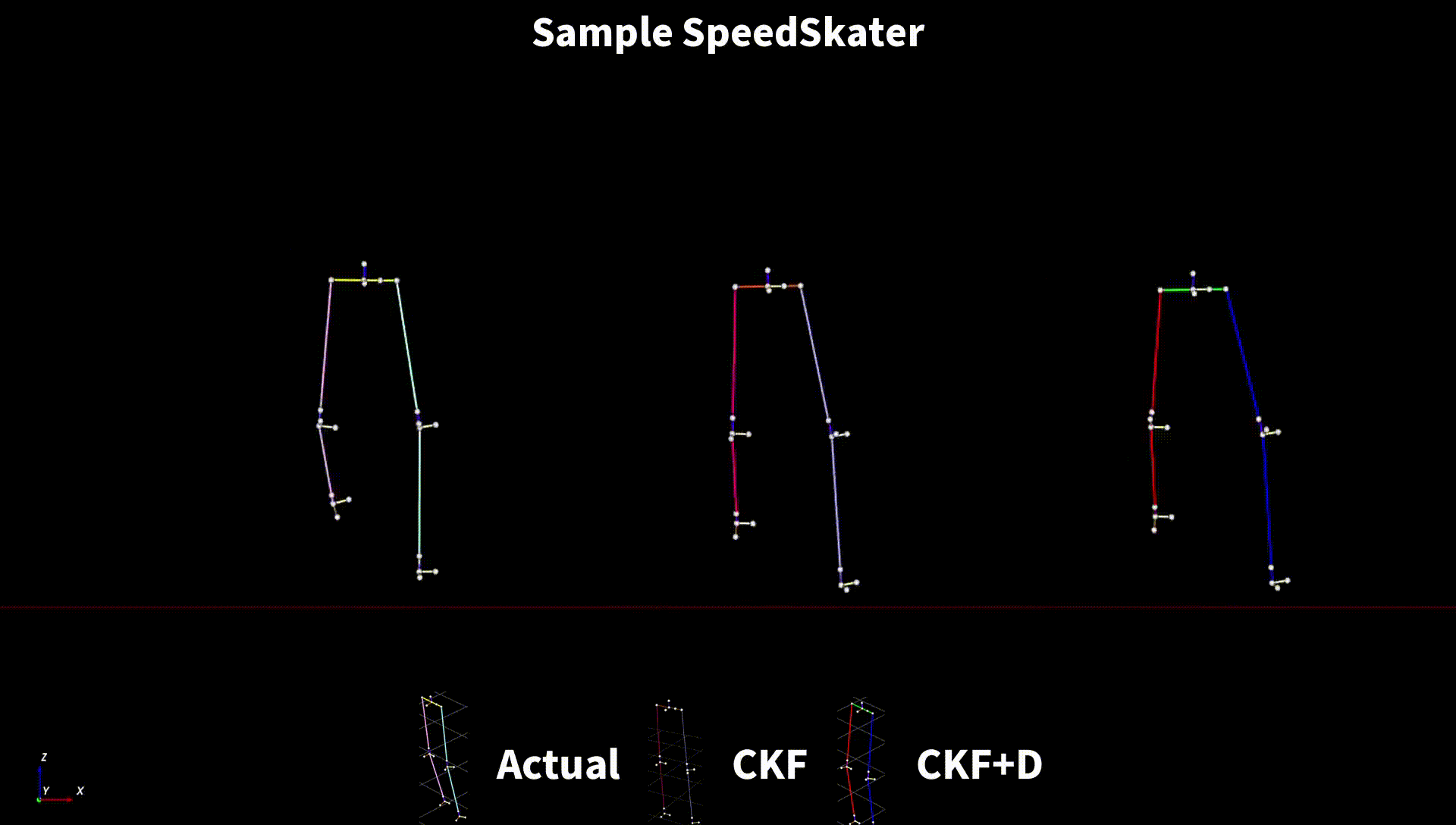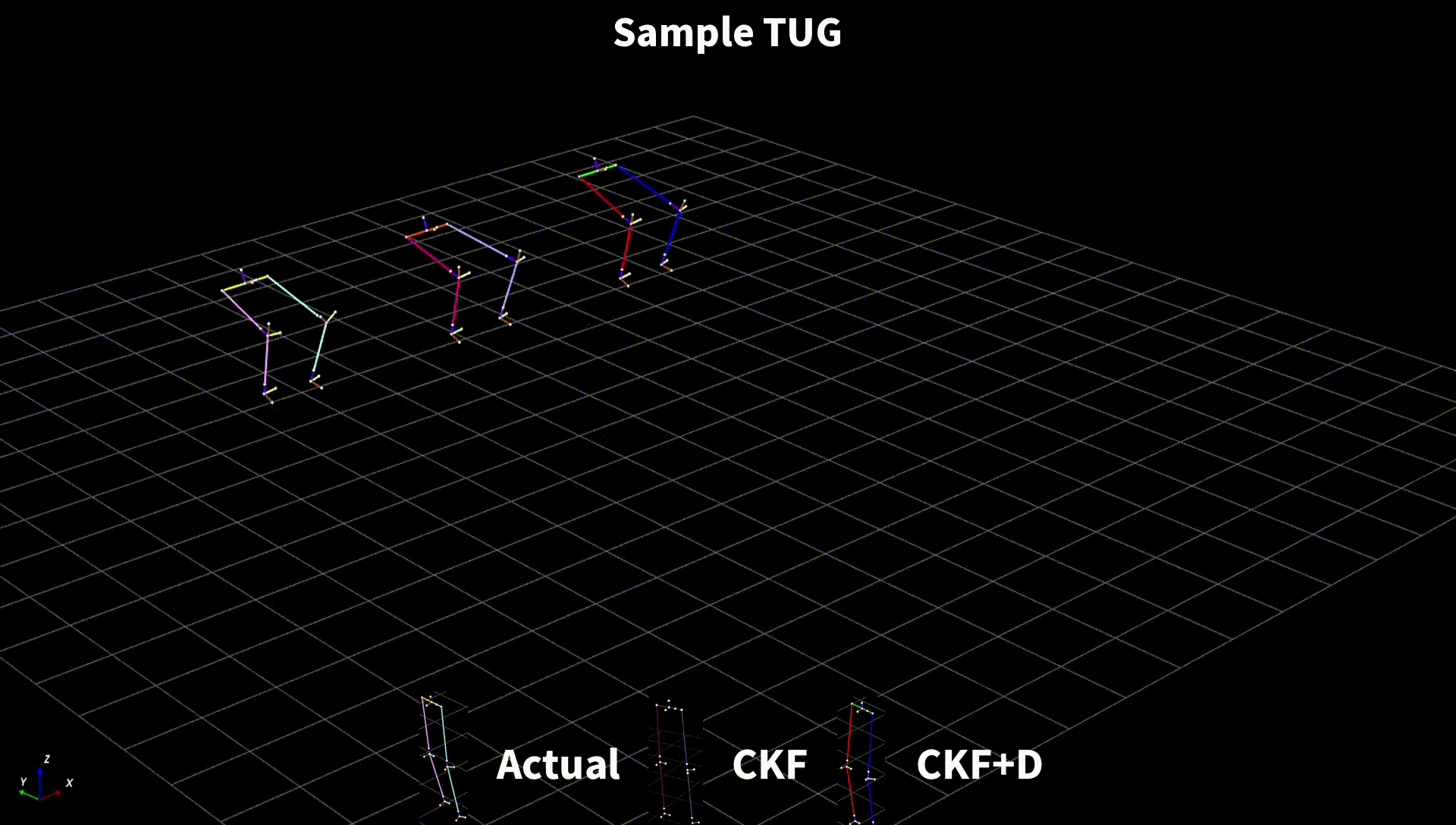## Sparse CKF+D - Varying $\sigma$

Simulated at different levels of distance measurement noise $\sigma$ (assumed gaussian). Useful from $\sigma \leq 0.1$ m for walking. Useful from $\sigma \leq 0.2$ m for dynamic movements.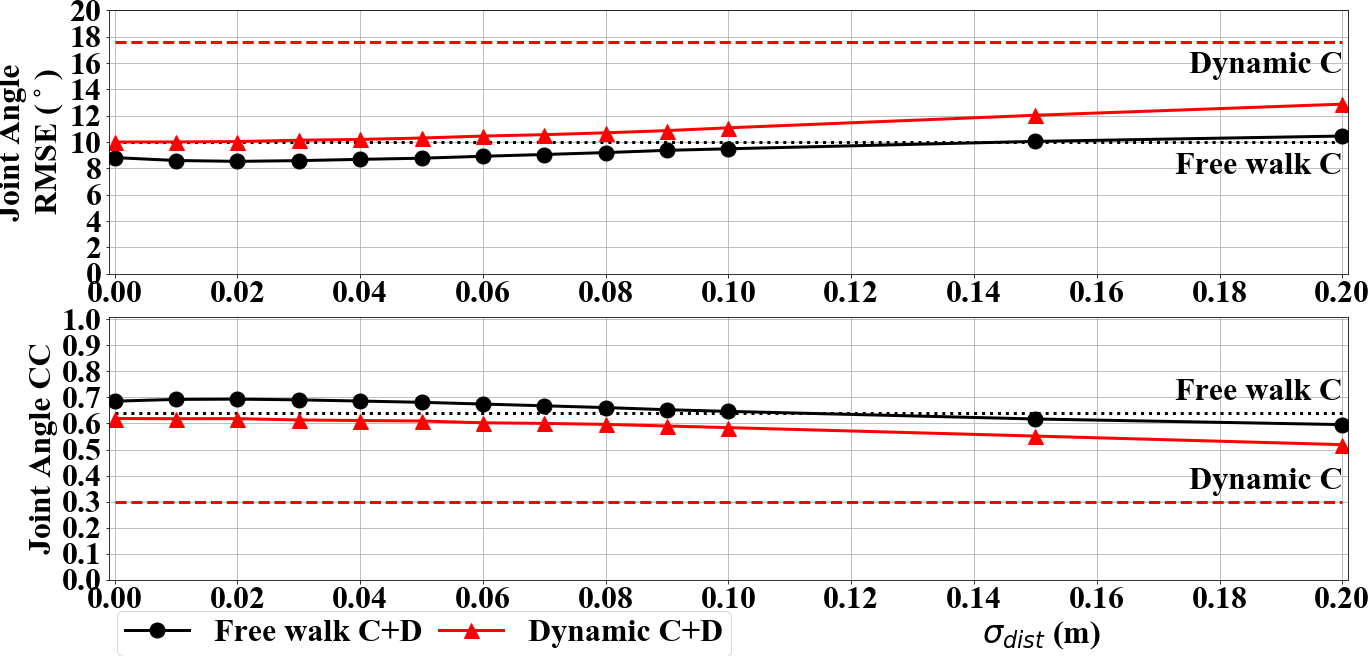## Conclusion & Future Work

• Adding distance measurement is indeed a promising approach
• Can be implemented using ultrasound or Ultrawideband (UWB) based sensors.
• Simulated distance measurement needs actual validation.
• Code at https://git.io/JvLCF
• Interesting to try with better models and tracking more segments.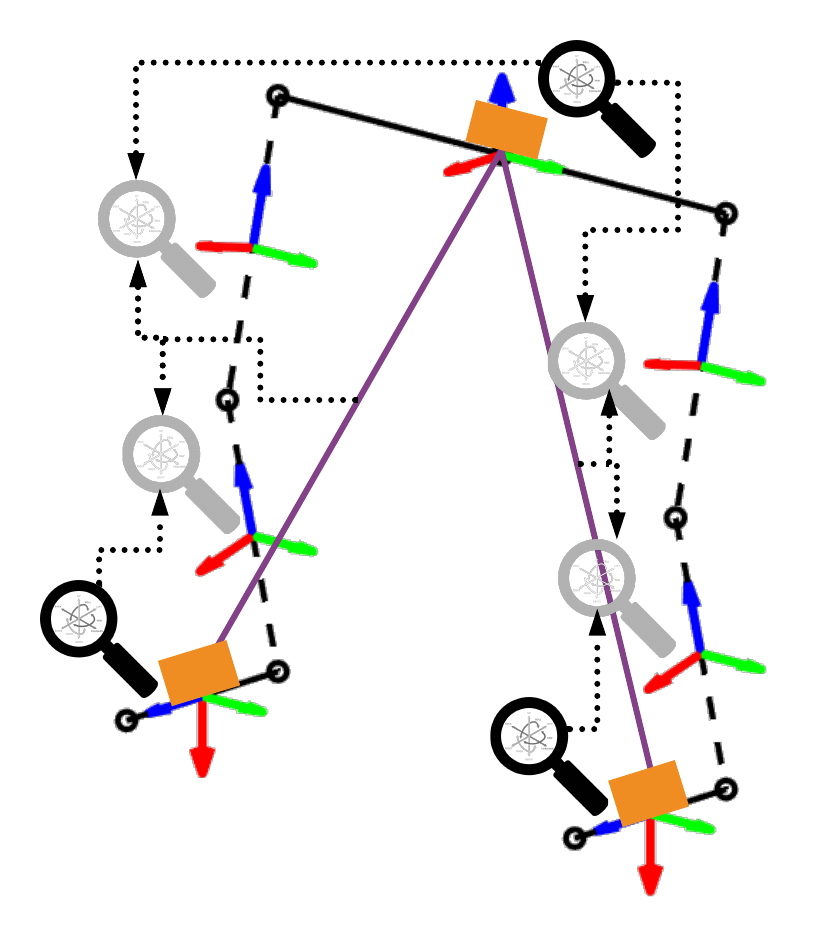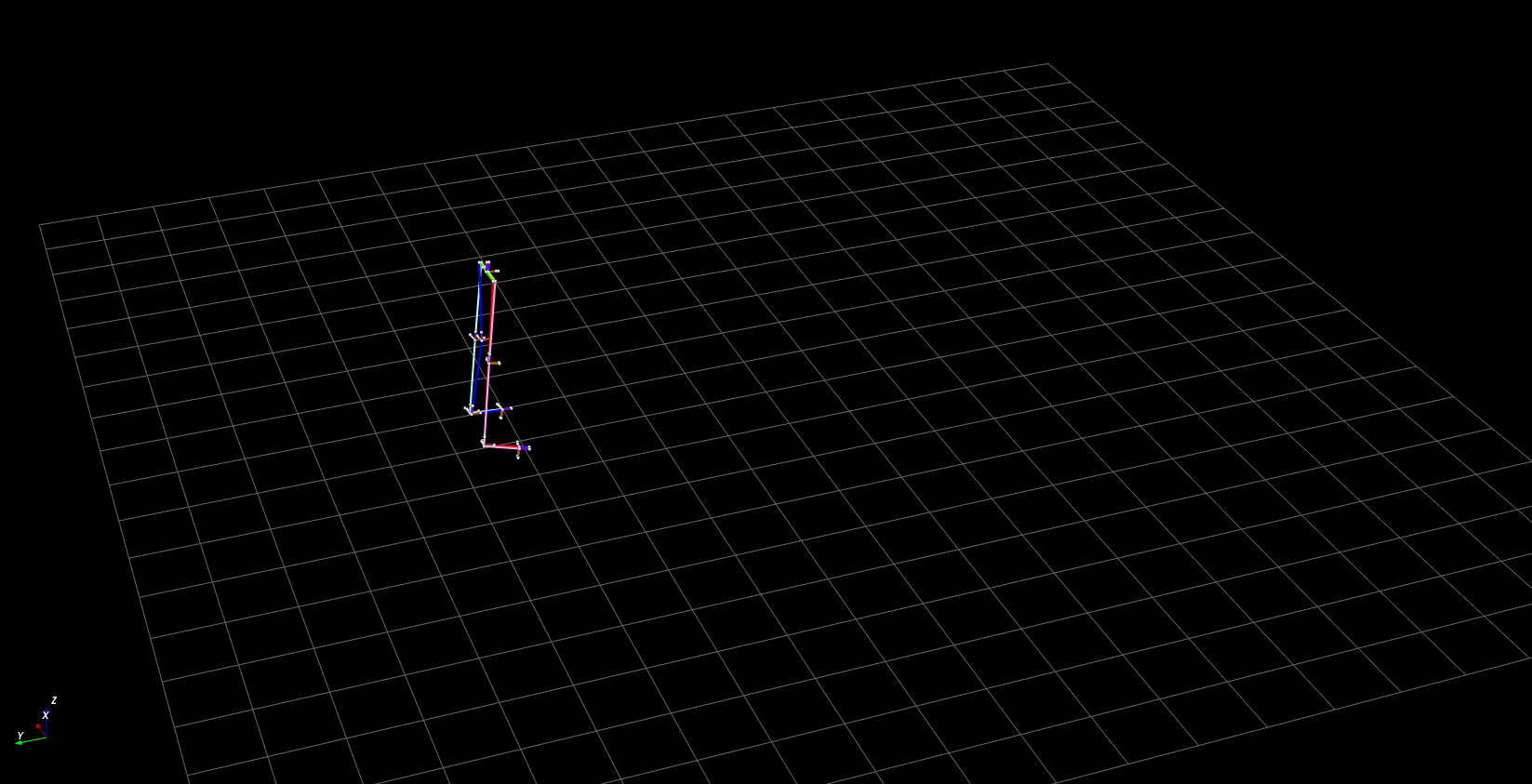Infer from distance.   Lie Group based 7 segment

## Appendix - CKF+D Quant. Results

Tested on actual IMU data + simulated distance measurement from Sparse CKF dataset (walking, jumping jacks, speedskater, TUG, jog).